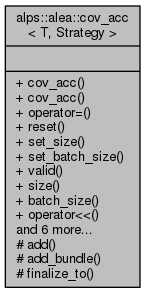ALPSCore reference
alps::alea::cov_acc< T, Strategy > Class Template Reference

#include <covariance.hpp>

Collaboration diagram for alps::alea::cov_acc< T, Strategy >:## Public Types

using value_type = T

using var_type = typename bind< Strategy, T >::var_type

using cov_type = typename bind< Strategy, T >::cov_type

using cov_matrix_type = typename eigen< cov_type >::matrix

## Public Member Functions

cov_acc (size_t size=1, size_t batch_size=1)

cov_acc (const cov_acc &other)

cov_accoperator= (const cov_acc &other)

void reset ()

void set_size (size_t size)

void set_batch_size (size_t batch_size)

bool valid () const

size_t size () const

size_t batch_size () const

cov_accoperator<< (const computed< T > &src)

cov_accoperator<< (const cov_result< T, Strategy > &result)

size_t count () const

cov_result< T, Strategy > result () const

cov_result< T, Strategy > finalize ()

const bundle< value_type > & current () const

const cov_data< T, Strategy > & store () const

## Protected Member Functions

void add (const computed< T > &source, size_t count)

void add_bundle ()

void finalize_to (cov_result< T, Strategy > &result)

## Friends

class batch_result< T >

## Detailed Description

### template<typename T, typename Strategy = circular_var> class alps::alea::cov_acc< T, Strategy >

Accumulator which tracks the mean and a naive covariance estimate.

Definition at line 21 of file covariance.hpp.

## Member Typedef Documentation

template<typename T, typename Strategy = circular_var>
 using alps::alea::cov_acc< T, Strategy >::cov_matrix_type = typename eigen::matrix

Definition at line 125 of file covariance.hpp.

template<typename T, typename Strategy = circular_var>
 using alps::alea::cov_acc< T, Strategy >::cov_type = typename bind::cov_type

Definition at line 124 of file covariance.hpp.

template<typename T, typename Strategy = circular_var>
 using alps::alea::cov_acc< T, Strategy >::value_type = T

Definition at line 122 of file covariance.hpp.

template<typename T, typename Strategy = circular_var>
 using alps::alea::cov_acc< T, Strategy >::var_type = typename bind::var_type

Definition at line 123 of file covariance.hpp.

## Constructor & Destructor Documentation

template<typename T , typename Str >
 alps::alea::cov_acc< T, Str >::cov_acc ( size_t size = 1, size_t batch_size = 1 )

Definition at line 75 of file covariance.cpp.

template<typename T , typename Str >
 alps::alea::cov_acc< T, Str >::cov_acc ( const cov_acc< T, Strategy > & other )

Definition at line 82 of file covariance.cpp.

## Member Function Documentation

template<typename T , typename Str >
 void alps::alea::cov_acc< T, Str >::add ( const computed< T > & source, size_t count )
protected

Definition at line 122 of file covariance.cpp.

template<typename T , typename Str >
 void alps::alea::cov_acc< T, Str >::add_bundle ( )
protected

Definition at line 186 of file covariance.cpp.

template<typename T, typename Strategy = circular_var>
 size_t alps::alea::cov_acc< T, Strategy >::batch_size ( ) const
inline

Returns number of data points per batch

Definition at line 150 of file covariance.hpp.

template<typename T, typename Strategy = circular_var>
 size_t alps::alea::cov_acc< T, Strategy >::count ( ) const
inline

Returns sample size, i.e., number of accumulated data points

Definition at line 159 of file covariance.hpp.

template<typename T, typename Strategy = circular_var>
 const bundle& alps::alea::cov_acc< T, Strategy >::current ( ) const
inline

Definition at line 167 of file covariance.hpp.

template<typename T , typename Str >
 cov_result< T, Str > alps::alea::cov_acc< T, Str >::finalize ( )

Frees data associated with accumulator and return result

Definition at line 161 of file covariance.cpp.

template<typename T, typename Strategy = circular_var>
 void alps::alea::cov_acc< T, Str >::finalize_to ( cov_result< T, Strategy > & result )
protected

Definition at line 169 of file covariance.cpp.

template<typename T, typename Strategy = circular_var>
 cov_acc& alps::alea::cov_acc< T, Strategy >::operator<< ( const computed< T > & src )
inline

Add computed vector to the accumulator

Definition at line 153 of file covariance.hpp.

template<typename T, typename Strategy = circular_var>
 cov_acc& alps::alea::cov_acc< T, Strategy >::operator<< ( const cov_result< T, Strategy > & result )

Merge partial result into accumulator

template<typename T , typename Str >
 cov_acc< T, Str > & alps::alea::cov_acc< T, Str >::operator= ( const cov_acc< T, Strategy > & other )

Definition at line 88 of file covariance.cpp.

template<typename T , typename Str >
 void alps::alea::cov_acc< T, Str >::reset ( )

Re-allocate and thus clear all accumulated data

Definition at line 96 of file covariance.cpp.

template<typename T , typename Str >
 cov_result< T, Str > alps::alea::cov_acc< T, Str >::result ( ) const

Returns result corresponding to current state of accumulator

Definition at line 152 of file covariance.cpp.

template<typename T , typename Str >
 void alps::alea::cov_acc< T, Str >::set_batch_size ( size_t batch_size )

Update the batch size and discard current batch

Definition at line 114 of file covariance.cpp.

template<typename T , typename Str >
 void alps::alea::cov_acc< T, Str >::set_size ( size_t size )

Update the size and discard all measurements, if any

Definition at line 106 of file covariance.cpp.

template<typename T, typename Strategy = circular_var>
 size_t alps::alea::cov_acc< T, Strategy >::size ( ) const
inline

Number of components of the random vector (e.g., size of mean)

Definition at line 147 of file covariance.hpp.

template<typename T, typename Strategy = circular_var>
 const cov_data& alps::alea::cov_acc< T, Strategy >::store ( ) const
inline

Return backend object used for storing estimands

Definition at line 170 of file covariance.hpp.

template<typename T, typename Strategy = circular_var>
 bool alps::alea::cov_acc< T, Strategy >::valid ( ) const
inline

Returns false if finalize() has been called, true otherwise

Definition at line 144 of file covariance.hpp.

## Friends And Related Function Documentation

template<typename T, typename Strategy = circular_var>
 friend class batch_result< T >
friend

Definition at line 183 of file covariance.hpp.

The documentation for this class was generated from the following files: